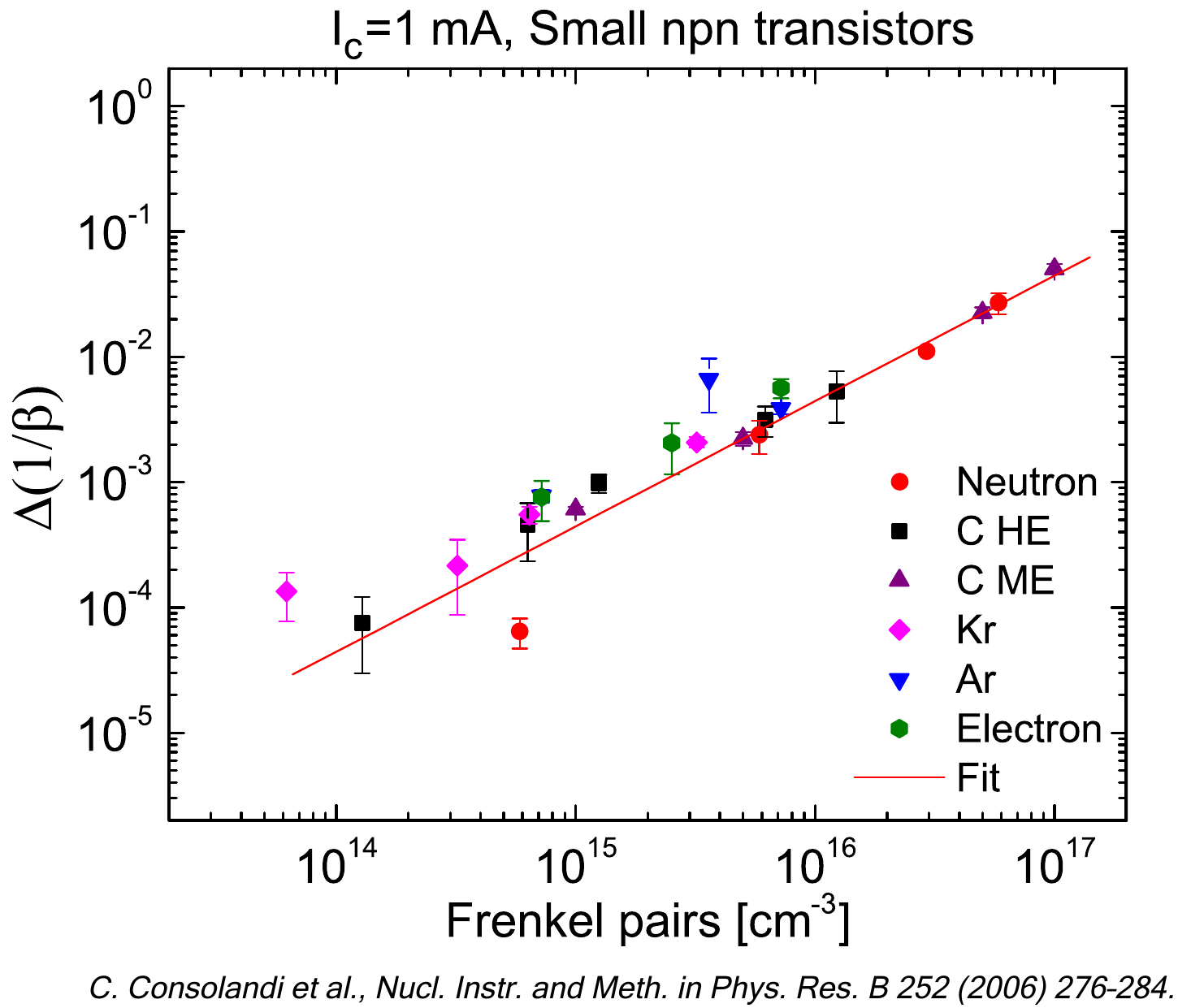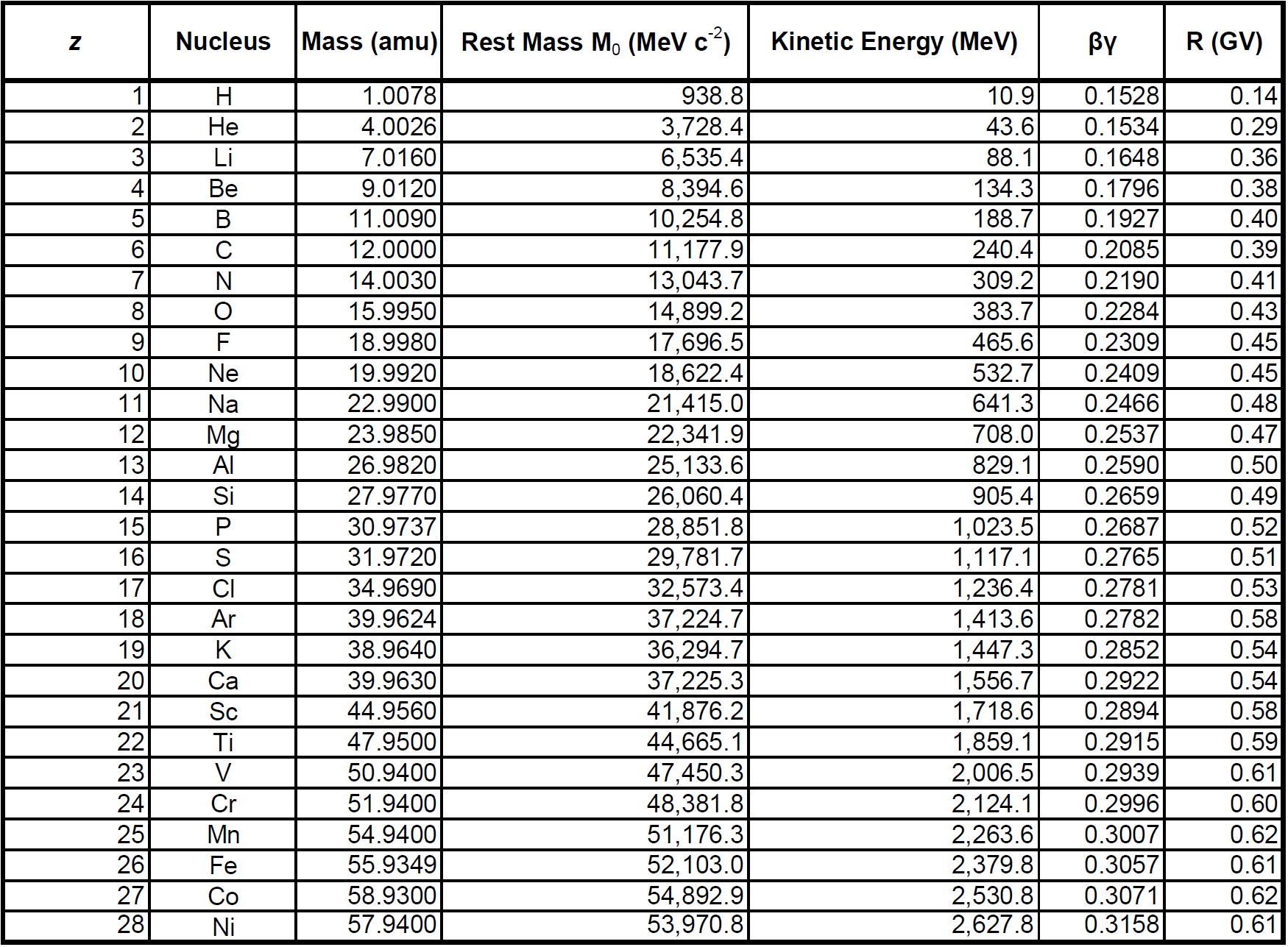SR-NIEL – 7 Screened Relativistic (SR) Treatment for NIEL Dose Nuclear and Electronic Stopping Power Calculator (version 10.2)The following table (for particles with 1< z <28 impinging perpendicularly to the absorber surface)  was obtained using the "SRIM Module.exe" included in SRIM 2013 code and implemeted in the sr-niel website here.

The kinetic energy thresholds were derived from SRIM data using an interpolation procedure.

The particle rigidity (R) is expressed in GV and is expressed as (e.g., see Eq. (8.94) at pag 642 of Leroy and Rancoita (2016)):where c is the speed of light, z is the particle atomic number, e is the electron charge and p is the incoming particle momentun in GeV/c.NOTE:

• an Al thickness of 50 mils corrisponds to 0.34 g/cm2
• the particle is assumed to imping perpendicularly to the absorber surface